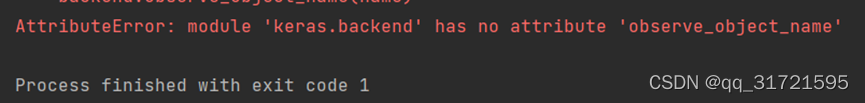# How to Solve keras load_model() Error

The problem is as follows:``self.model = load_model(path_to_model, custom_objects={'f1': f1})``

Solution:

``from keras.models import load_model``
``from tensorflow.keras.models import load_model``